### Evolutionary Dynamics

Ken Webb 2010-03-23T13:05:53Z

The 2006 book Evolutionary Dynamics - Exploring the equations of life http://www.ped.fas.harvard.edu/ by Martin Nowak includes numerous detailed examples. It was straight forward to implement the basic models in the Spatial Games http://www.univie.ac.at/virtuallabs/Introduction/sd.html chapter using Xholon. The following results from the Xholon implementation roughly match the images included in the book (page 150-151).

b = 1.10

b = 1.15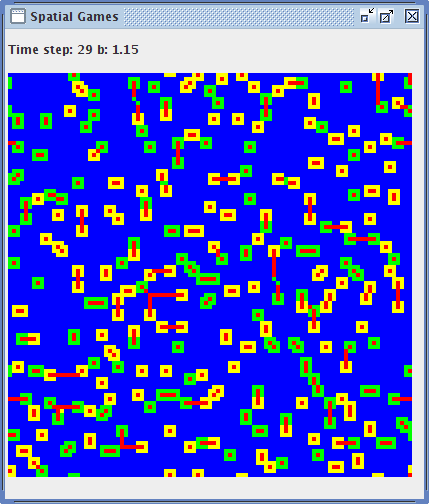b = 1.24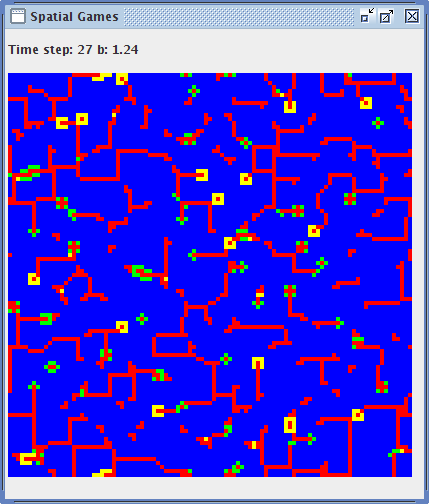b = 1.35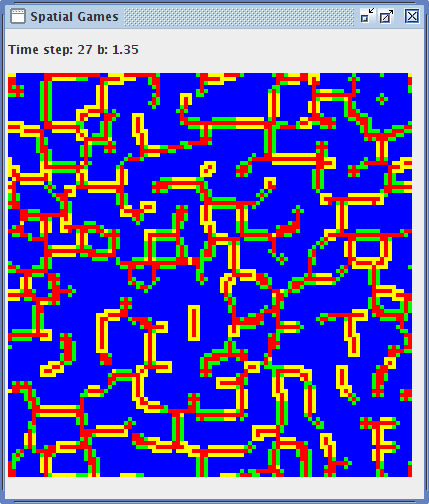b = 1.55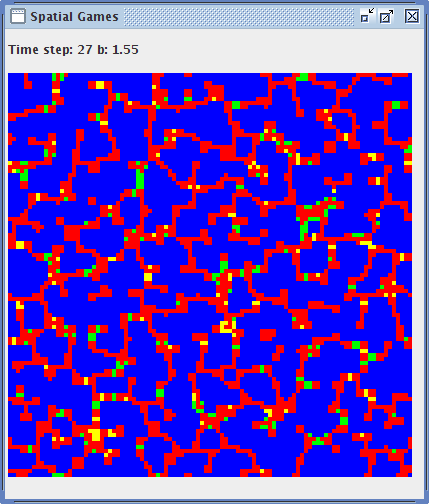b = 1.65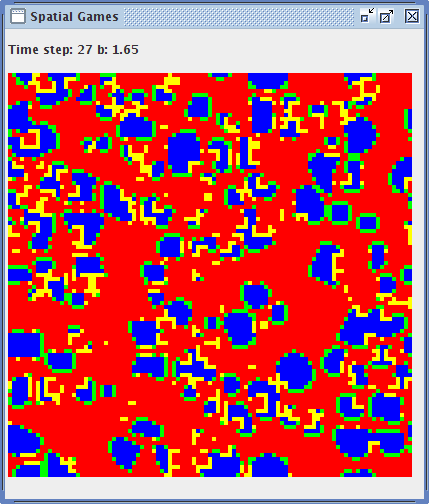b = 1.70Also in chapter 9, the book goes on to describe what happens if there is just a single defector rather than a random assortment of cooperators and defectors. The t=30 image matches exactly the image on page 158 of the book.

b = 1.65, single defector, t = 30b = 1.65, single defector, t = 2402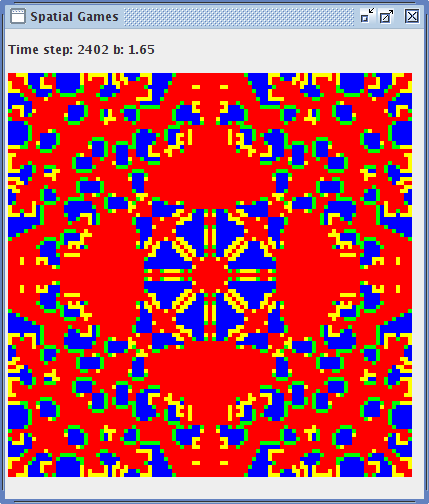The Xholon GUI, which is used to control the simulation and view the state of the objects in the grid, is shown below for the single defector t=2402 run.